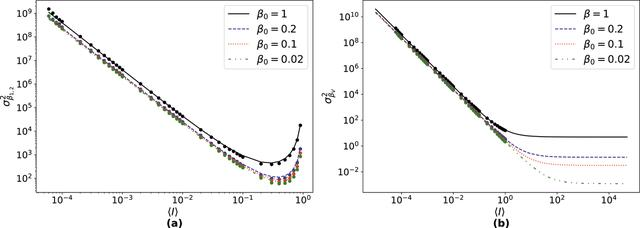disable zoom     view article Figure 4 (a) Variance of β1,2 as a function of 〈I〉 as computed using equation (12)(solid lines) and simulated values (dots). The variance decreases with increasing 〈I〉 for low signals and then increases again as 〈I〉 approaches 1. (b) Variance of βV as a function of 〈I〉 as computed using equation (13)(solid lines) and simulated values (dots). Note thatsaturates at high 〈I〉.JOURNAL OFSYNCHROTRONRADIATION
ISSN: 1600-5775
Volume 30| Part 1| January 2023| Pages 11-23